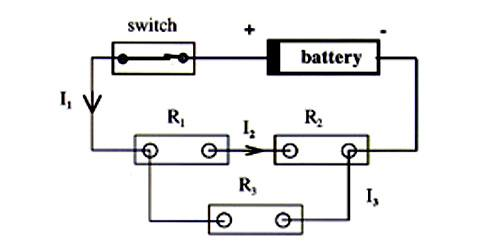# Kirchhoff's rules for resistors in parallel

coleman221

## Homework StatementUse Kirchoff's rules to determine I1, I2 and I3 for the following circuit:

Use the following values:

ε = emf = 9 V
r = internal resistance = 1.5
R1 = 5
R2 = 15
R3 = 13.75

## The Attempt at a Solution

I found I1 to be .9328 by adding all the resistors together (taking into account that r3 was in parallel to r1 and r2) and adding the resistance of the battery.

I've been trying to use 0=ε-I1r-IR1-IR2
0=ε-I1r-I3R3
I1=I2+I3

I've done similar problems but this is for a lab instead of homework so its written in different a way (and they like to throw in things we haven't learned yet in lecture) so i think I'm having trouble visualizing where the currents are splitting and what parts are equivalent.